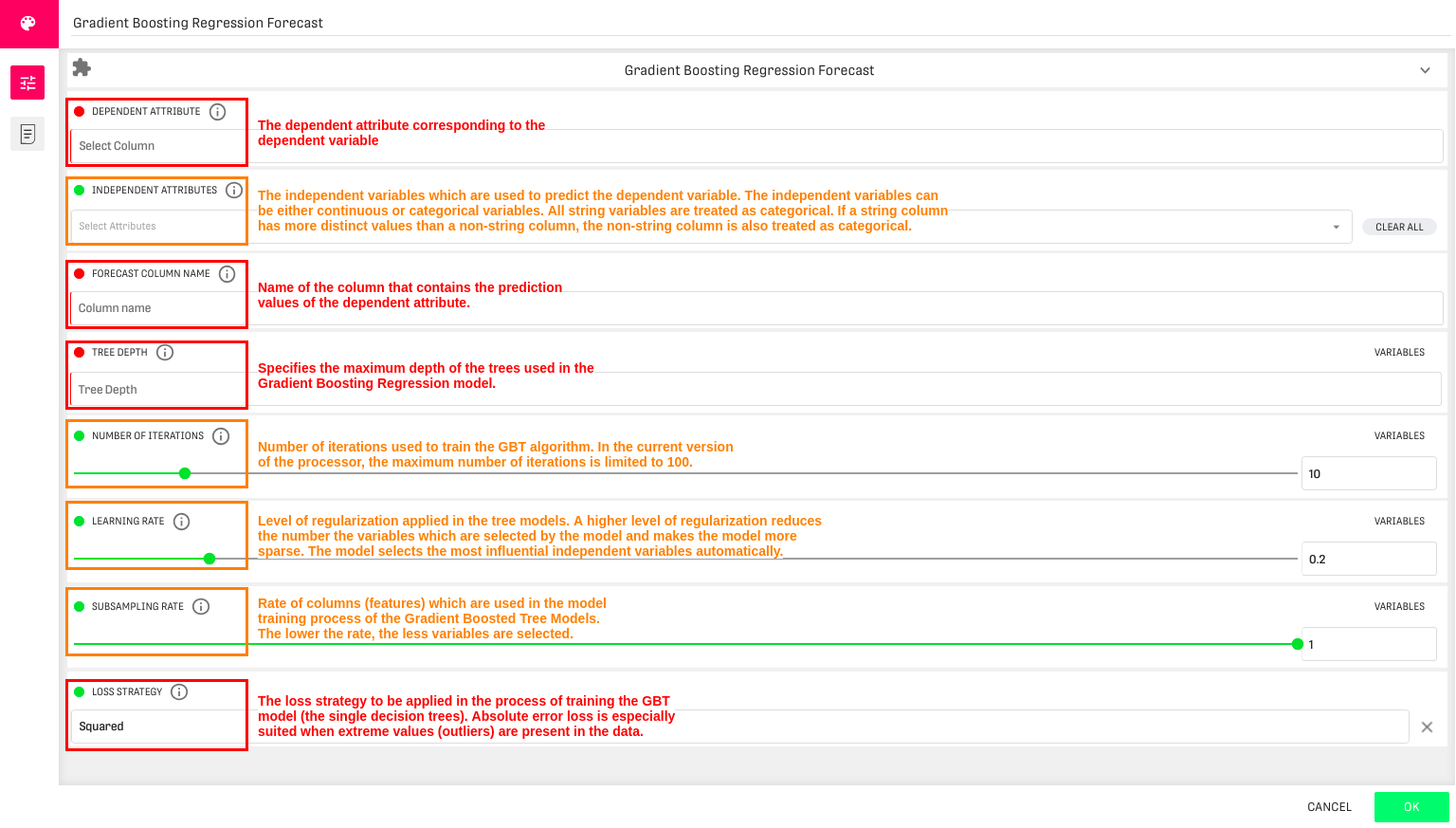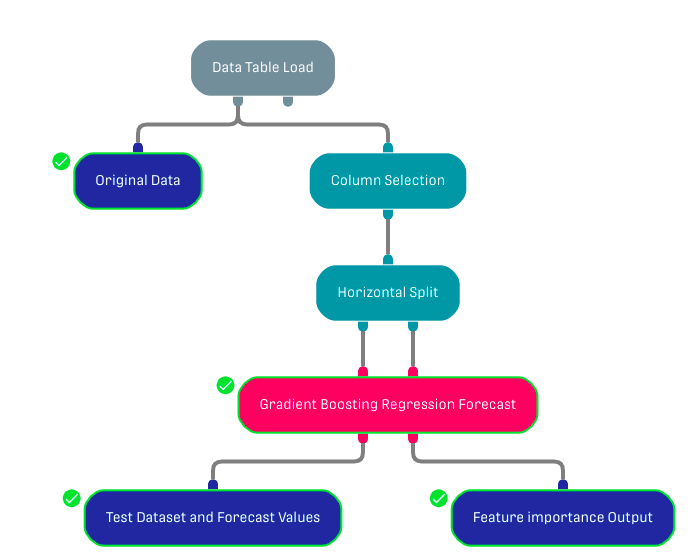# Gradient Boosting Regression Forecast Processor

Modified on Tue, 30 Nov 2021 at 04:37 PM

# Overview

Predicts the value of a dependent variable using Gradient Boosted Trees (GBTs) in a regression setting.

Gradient boosting is known to be one of the leading ensemble algorithm. It uses gradient descent method to optimize the loss function.

# Input

The processor requires two input datasets. The first input port (the one on the left) corresponding to the training dataset (this data should be already labeled). The second input port (the one on the right) corresponding to the test dataset.

The training and the test datasets should have the same schema.

# ConfigurationThe dependent variable has to be numeric and continuous. The independent variables can either be continuous or categorical.

# Output

The Gradient Boosting Regression Forecast Processor returns two different output tables:

• Test Dataset and Forecast Values: The input test data is forwarded along with three new columns: The created forecast column (the name of the column is specified in the third configuration field) and two new columns with probabilities for the values 0 and 1 (the name for these columns is specified in the last configuration field).
• Feature Importance Output: Returns the variable importance ranking for all independent variables within a two column table. It shows which of the independent variables were most important in predicting the dependent variable.

In addition, a bar chart with the feature importance result is shown in the result tab within the Gradient Boosting Classification Forecast Processor.

# Example

In this example, the used data table contains YouTube videos relative information (total likes, dislikes, etc.). The processor of focus is used to forecast the number of comments.

##In the previous workflow, the Column Selection processor is used to select the dependent and independent columns only. Then the dataset is split into a training and test dataset and inserted in the target processor by a Horizontal Split processor.

# Related Articles

Decision Tree Regression Forecast

Decision Tree Classification Forecast

Random Forest Classification Forecast Processor

Random Forest Regression Forecast Processor